## Solving Polynomial Inequalities by GraphingThis section assumes that you have access to a graphing calculator or some other graphing program.

Let's suppose you want to solve the inequality

x2-1<0.

Here is the graph of the function f(x)=x2-1: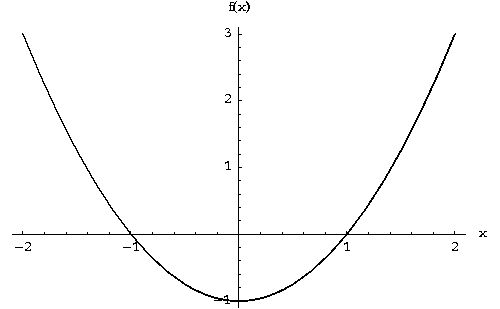A given x will solve the inequality if f(x)<0, i.e., if f(x) is below the x-axis. Thus the set of our solutions is the part of the x-axis indicated below in red, the interval (-1,1):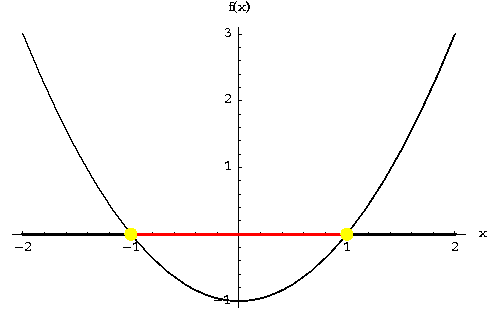If we want to see the solutions of the inequality

x2-1>0,

that's just as easy. Now we have to pick all values of x for which f(x)=x2-1 is above the x-axis. As you can see, we obtain as solutions the set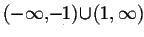, indicated below in blue.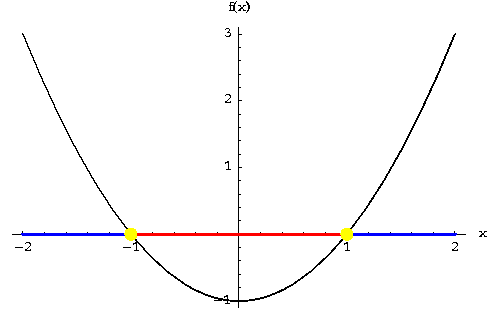Note the pivotal role played by the "yellow dots", the x-intercepts of f(x).

f(x) can only change its sign by passing through an x-intercept, i.e., a solution of f(x)=0 will always separate parts of the graph of f(x) above the x-axis from parts below the x-axis. This property of polynomials is called the Intermediate Value Property of polynomials; your teacher might also refer to this property as continuity.

Let us consider another example: Solve the inequality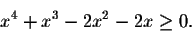Here is the graph of the function f(x)=x4+x3-2x2-2x>0: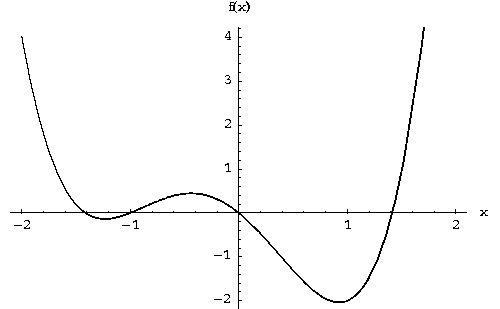A given x will solve the inequality if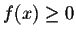, i.e., if f(x) is above the x-axis. Thus the set of our solutions is the part of the x-axis indicated below in blue, the union of the following three intervals: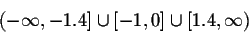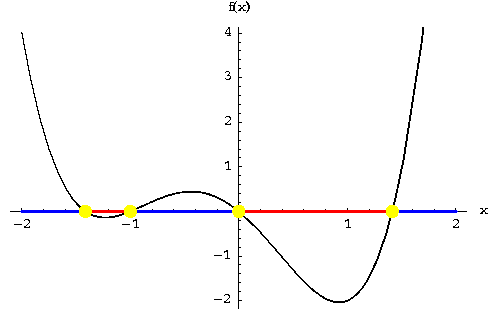The (finite) endpoints are included since at these points f(x)=0 and so these x's are included in our quest of finding the solutions of.

Our answer is approximate, the endpoints of the intervals were found by inspection; you can usually obtain better estimates for the endpoints by using a numerical solver to find the solutions of f(x)=0. In fact, as you will learn in the next section, the precise endpoints of the intervals are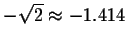, -1, 0 and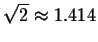.

Two more caveats: The method will only work, if your graphing window contains all x-intercepts. Here is a rather simple-minded example to illustrate the point: Suppose you want to solve the inequality

x2-10x<0.

If your graphing window is set to the interval [-5,5], you will miss half of the action, and probably come up with the incorrect answer: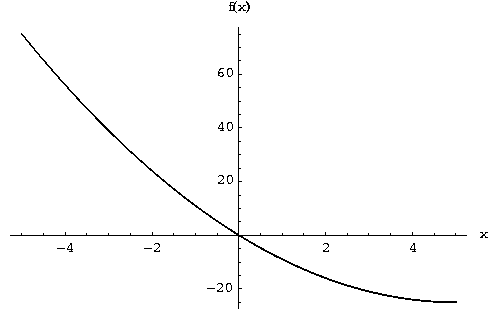To find the correct answer, the interval (0,10), your graphing window has to include the second x-intercept at x=10: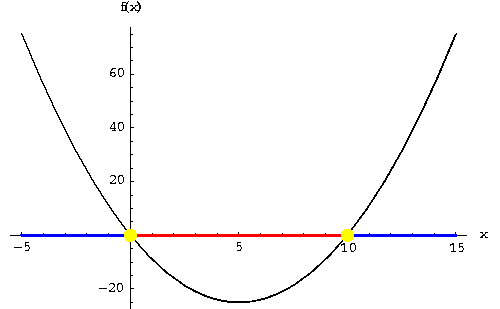Here is another danger: Consider the three inequalities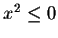,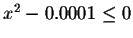and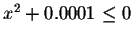. If you do not zoom in rather drastically, all three graphs look about the same: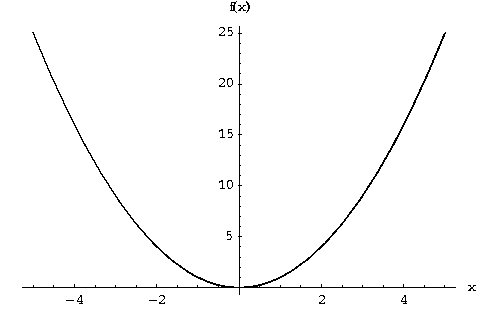Only zooming in reveals that the solutions to the three inequalities show a rather different behavior. The first inequality has a single solution, x=0. (This also illustrates the fact that a function f(x) does not always change sign at points where f(x)=0.)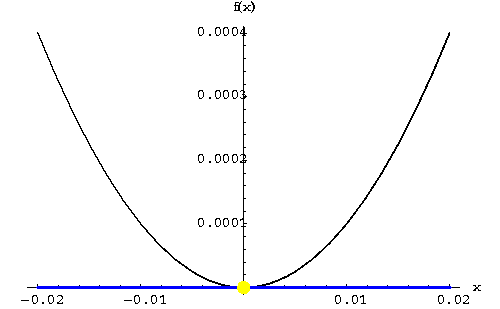The second inequality,, has as its solutions the interval [-0.01,0.01]: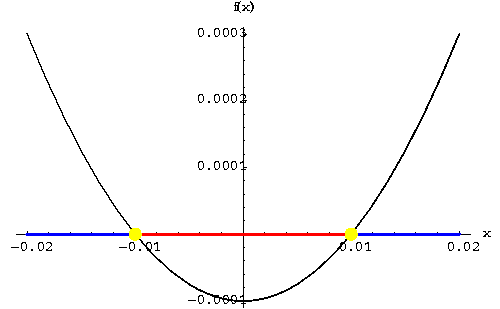The third inequality,, has no solutions: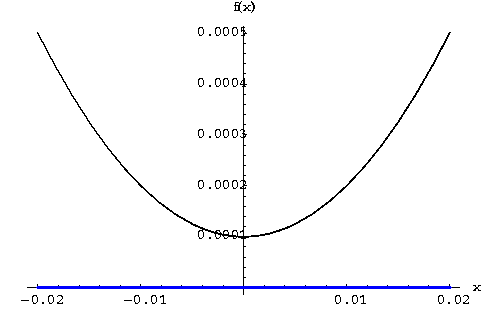[Back] [Next]
[Algebra] [Trigonometry] [Complex Variables]
[Calculus] [Differential Equations] [Matrix Algebra]S.O.S MATHematics home page

Do you need more help? Please post your question on our S.O.S. Mathematics CyberBoard.Helmut Knaust